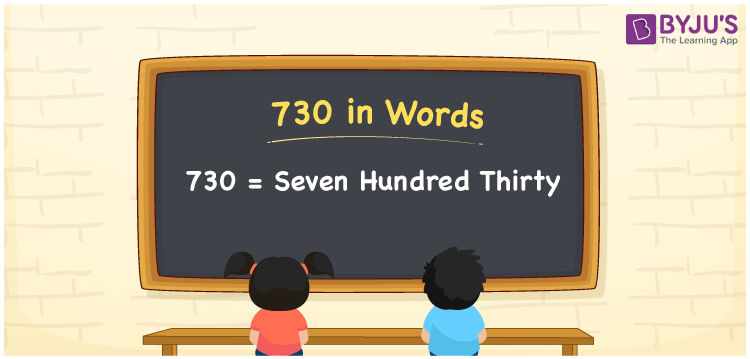# 730 in Words

730 in words can be written as Seven Hundred Thirty. If you buy a pair of slippers for Rs. 730, then you can say that “I bought a pair of slippers for Seven Hundred Thirty Rupees”. Numbers in words can be well grasped in this article as it plays an important role in the higher education levels of students. Hence, the number 730 can be read as “Seven Hundred Thirty” in English.

 730 in words Seven Hundred Thirty Seven Hundred Thirty in Numbers 730

## 730 in English Words## How to Write 730 in Words?

This section provides an in depth idea of the place value chart of 730 which is crucial for the exams. Check the table below and understand the concept with ease.

 Hundreds Tens Ones 7 3 0

The expanded form of 730 is provided below:

7 x Hundred + 3 × Ten + 0 × One

= 7 x 100 + 3 x 10 + 0 x 1

= 700 + 30

= 730

= Seven Hundred Thirty

Therefore, 730 in words is written as Seven Hundred Thirty.

730 is a natural number that precedes 731 and succeeds 729.

730 in words – Seven Hundred Thirty

Is 730 an odd number? – No

Is 730 an even number? – Yes

Is 730 a perfect square number? – No

Is 730 a perfect cube number? – No

Is 730 a prime number? – No

Is 730 a composite number? – Yes

## Frequently Asked Questions on 730 in Words

Q1

### How do you write the number 730 in words?

730 in words is Seven Hundred Thirty.
Q2

### Find the value of 800 minus 70.

The value of 800 minus 70 is 730. Therefore, 730 in words is Seven Hundred Thirty.
Q3

### Is 730 a perfect square?

No, 730 is not a perfect square as it cannot be expressed as the product of two integers which are equal.